# Quiz 2: The Balance Sheet

1. Prepare journal entries of April 2013 as below: Journal entries For the month of April 2013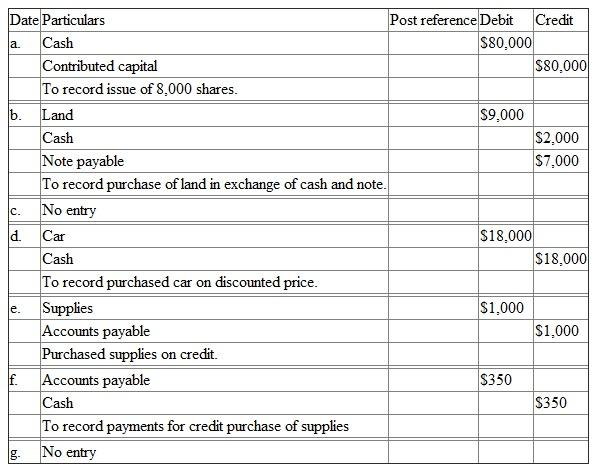a. Capital of \$80,000: Cash is an asset and increases, therefore debited. It is in exchange of capital. Contributed capital is a liability of the company. It increases, therefore credited. b. Land of \$9,000: Land is an asset and increases, therefore debited. Paid cash of \$2,000 reduces cash amount, therefore credited. The credit amount of \$7,000 creates liability of the company. Since the liability increases, accounts payable is credited. c. Since the work of employee is not yet started, there should not be any entry in this month. d. Bought car of \$18,000: Cash is an asset and decreases, therefore credited. Car is an asset and increases, therefore debited. e. Supplies of \$1,000 bought on credit: Increasing supplies increases assets, therefore debited. Since the payment is not made, it creates liability. Therefore, accounts payable is credited. f. Payment of \$350 to accounts payable: Payment of cash reduces cash. Therefore, cash is credited. Since accounts payable is paid off, it reduces liability. Therefore, accounts payable is debited. g. Sale of individual shares of \$300: This is the personal transaction of a stockholder. It has no connection with business. Therefore, there should not be any entry. 2. T-account: This is the individual account detail of each head. It starts from opening balance and ends with closing balance. In between there are so many transactions during the accounting period. Cash: This is an asset. Cash increases if debited and decreases if credited. T-account of cash is as below: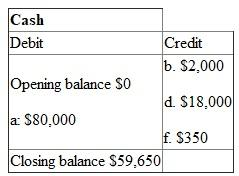The debit cash balance is \$59,650. It means the company has \$59,650 in hand at the end of the month. Land: This is an asset and becomes property of a company. It increases if debited and decreases if credited. T-account of Land is as below: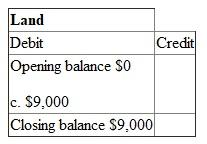The value of land at the end of month is \$9,000. Contributed capital: This is the portion of company's shareholdings. This is a liability of the company. It increases if credited and decreases if debited. T-account of contributed capital is as below: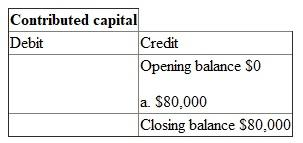The ownership right in the company is \$80,000. Note payable: This is the signing amount of borrowing money. This is a liability of the company. It increases if credited and decreases if debited. T-account of note payable is as below: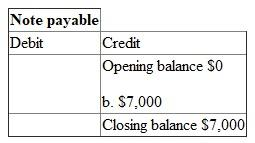This is a long term note payable, since the payment will be made after 1 year. Car: This is an asset and becomes property of a company. It increases if debited and decreases if credited. T-account of Car is as below: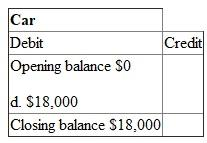The value of car at the end of month is \$18,000. Supplies: This is the stock account. It is an asset. It increases if debited and decreases if credited. T-account of supplies is as below: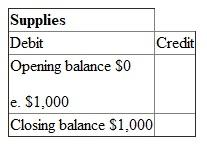The closing stock is \$1,000. Accounts payable: This is the due amount to suppliers. It indicates company's obligation to pay. Therefore this is a liability. It increases if credited and decreases if debited. T-account of accounts payable is as below: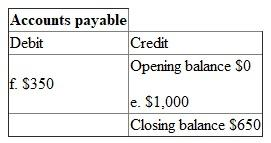Since it is a liability, the opening and closing balance should appear in the credit side. 3. Balance sheet: This is the company's financial situation till date. Assets and liabilities are recorded in the balance sheet. The aggregate of both sides will tally certainly. Asset: This is the amount of property of a company as on date. It has two parts-current asset and fixed asset. The liquidate assets are current assets. Heavy assets are known as fixed assets. Liability: This is the amount of obligation of a company as on date. The liabilities that met within a year are current liabilities Prepare the balance sheet below at the end of April 2013: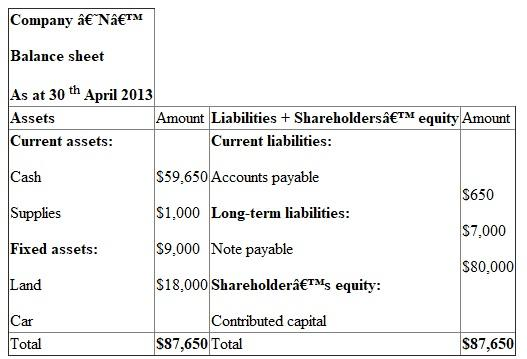Shareholders' equity: This is the ownership right of investors in a business. The above balance sheet is tallying. It indicates correctness of doing the solutions. 4. Current ratio: This is the ratio of current asset and current liability. Current asset means property of short period. Current liability means debt of short period. Calculate the current ratio of the company at the end of 30-04-2013 below:= 93.30 Current ratio is 93.30. It indicates of having \$93.30 current assets for each \$1 liability. Since the ratio is more than 2, the company's ability of payment is excellent.

There is no answer for this question

Match each of the terms with its related definition: • Transaction: A transaction occurs on account of undertaking a business activity, and it has effect on assets, liabilities and the stockholder's equity. • Separate entity assumption: Separate entity assumption states that the owner of the business is separate from the corporation. • Balance sheet: Balance sheet is constructed based on the basic accounting equation,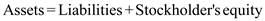. Both debit column and the credit column should match for a balance sheet. • Liabilities: Liabilities are part of balance sheet. These are the amounts which the business owes to its creditors. •: An accounting equation states what a company owns and what it owes. An asset is a resource which is owned by the company. Liability is the money which the company owes to its creditors. Further, equity refers to the amount invested by the owners of the company. Hence, it is the basic accounting equation. • Current assets: Assets which can be easily converted into cash within one year are called current assets. • Notes payable: Notes payable are nothing but the accounts payable. But they can be outstanding for more than one year and interest is charged on them. • Duality of effect: This states that every transaction effects both debit and credit balance. • Retained earnings: Retained earnings are profits earned by the company that are ploughed back into company without distributing to stockholders. • Debit: A debit increases assets, and decreases liabilities and stockholder's equity.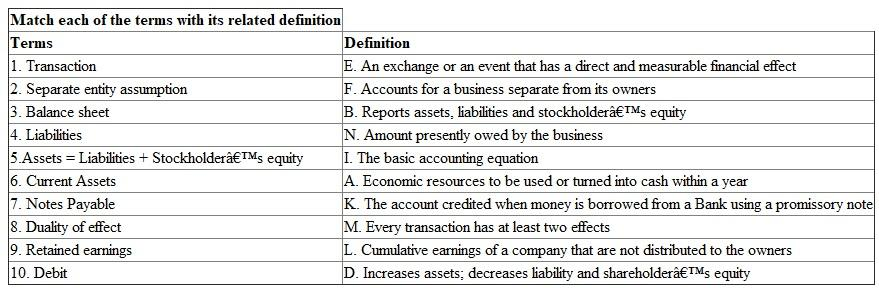There is no answer for this question

There is no answer for this question

There is no answer for this question

There is no answer for this question